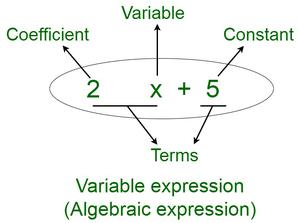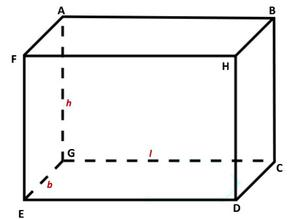GeeksforGeeks App
Open AppBrowser
Continue

# Calculate the volume of a cuboidal box whose dimensions are 5x × 3x2 × 7x4

Algebraic expressions are the equations that are obtained when operations such as addition, subtraction, multiplication, division, etc. are operated upon by any variable. Many day-to-day life problems can be manipulated and easily solved by using algebraic Expression. For example, suppose a situation is given in which there are two girls A and B. The amount of money A has, is 3 more than 2 times money owned by B. If one assumes that money owned by B is x then, money owned by A can be shown as 2x + 3. Thus, one can say that 2x + 3 is an example of an algebraic expression.

### Components of an algebraic expression

There are different components of an algebraic expression. Below are 4 components mentioned that are seen in the algebraic expression. Let’s learn about them in brief,

• Terms: These are products of coefficient and variable or only constant.
• Variables: Variables are symbols whose values can vary. These are usually denoted by letters of the alphabet.
• Coefficients: Coefficients of a variable is the number written along with the variable in the term.
• Constants: Constants are numbers whose value cannot be changed.

Given below image clearly shows the above components of an algebraic expression.Example 1: Solve the following algebraic equation 2x + 5 = 10{(x / 2 ) – 4}.

Solution:

2x + 5 = 10{(x/2) – 4}

2x + 5 = 10 × (x/2) – 10 × 4

2x + 5 = 5x – 40

Multiply both sides by -1 and bringing like terms together.

5x – 2x = 40 + 5

3x = 45

x = 15

So, the value of x is 15.

Example 2: Solve and find the values of x: 2x2 + 5x + 3 = 0

Solution:

2x2 + 5x + 3 =0

Splitting the middleterm, 2 +3 = 5  and 2 × 3 = 6 .

2x2 + 2x + 3x + 3 = 0

2x (x + 1) + 3 (x + 1) = 0

(2x + 3) (x + 1) = 0

2x + 3 = 0 and x+1=0

x = -3/2 and x = -1

### Volume of Cuboid

A cuboid is a 3-Dimensional object which has length, breadth, height. Cuboid can be formed by placing rectangles one above the other. By placing rectangles one on the other it gains an extra height.So, the volume of cuboids can be calculated using formulae,

Volume of cuboid (V) = l × b × h

Where, l = length,

h = height

### Calculate the volume of a cuboidal box whose dimensions are 5x × 3x2 × 7x4.

Solution:

Step1: Take a look at the options already given in the problem statement.

Given:

length = 5x

height = 7x4

Step2: Calculate the volume of cuboidal box by using formulae,

V = l × b × h

Step3: By putting values in the above formulae.

V = 5x × 3x2 × 7x4

Step4: Coefficients are multiplied and powers are added because base is same. So, After simplification,

V = 105x7

So, Volume of given cuboidal box is 105x7 cubic units.

Now, for any given x volume can be easily calculated.

For example, for x = 2 ,

V = 105 × (2)7

V = 105 × 128

V = 13440 cubic units.

### Sample Problems

Question 1: A box has a length of 2m, breadth of 3m, and height of 5m. Find the volume of the box.

Solution:

l = 2m

b = 3m

h = 5m

V = 2m × 3m × 5m

V = 30m3

So, volume of given box is 30 m3.

Question 2: A rectangular sheet of length 10cm and breadth 25cm is placed one above the other to form a pile of height 1cm. Find the volume of the pile generated.

Solution:

l = 10cm

b = 25cm

h = 1cm

V = 10cm × 25cm × 1cm

V = 250cm

So, volume of the given pile is 250cm3.

My Personal Notes arrow_drop_up
Related Tutorials